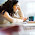## Tuesday, 10 December 2013

### Decision Tree - rpart

There is a number of decision tree algorithms available. In this blog, I am describing the rpart algorithm which stands for recursive partitioning and regression tree. This algorithm allows for both regression and classification, and handles the data relatively well when there are many categorical variables.

Decision trees may lack accuracies compared to other more sophisticated techniques, but its main advantages include less restrictions during analysis and easier interpretation of its outputs even by non-technical experts such as managements or operational staff.

This algorithm requires 'rpart' package in R, and rpart() function is used to build a tree as seen in the below examples.

### Classification Tree

When you have a categorical variable as y value (or target), the tree is a classification tree and you can write the function as below.

RP1 <- rpart(Species ~ ., iris)

This would produce a tree that looks like below.

## n= 150 ## ## node), split, n, loss, yval, (yprob) ## * denotes terminal node ## ## 1) root 150 100 setosa (0.33333 0.33333 0.33333) ## 2) Petal.Length< 2.45 50 0 setosa (1.00000 0.00000 0.00000) * ## 3) Petal.Length>=2.45 100 50 versicolor (0.00000 0.50000 0.50000) ## 6) Petal.Width< 1.75 54 5 versicolor (0.00000 0.90741 0.09259) * ## 7) Petal.Width>=1.75 46 1 virginica (0.00000 0.02174 0.97826) *

In this example, where the target variable is a categorical variable, the decision tree will look at probabilities of the categorical values of the target variable at each node and leaf, and display the one with the highest probability.

In this representation of the tree, leaf is the terminal node where no further splits are made, and is denoted with an asterisk (*) sign. Node is a point in the branch where a split is made.

At each leaf and node, the following output values are displayed:
• condition of the split (e.g. Petal.Length< 2.45)
• number of data points belonging to the node/leaf
• number of loss (i.e. number of misclassification) at the node/leaf
• target value of the highest probability at the node/leaf
• in brackets, probability scores of each target value at the node/leaf
To view the tree in a graphical representation, use the following functions.

plot(RP1) text(RP1, cex = 0.8)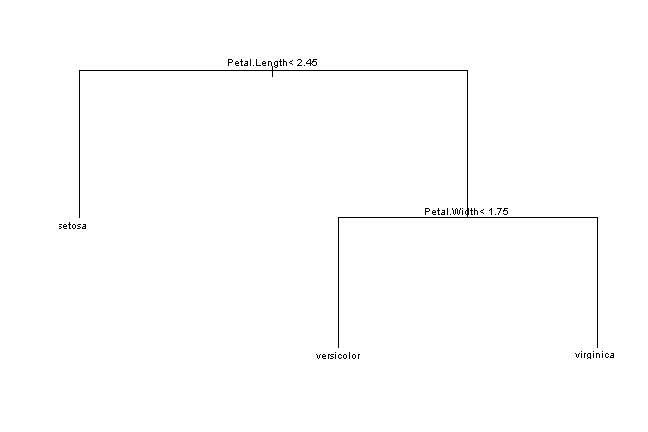In the above graphical representation, the data points that satisfy the splitting condition at the node moves to the branch on the left.

### Regression Tree

When you set a numerical variable as y value (or target), the tree is a regression tree and you can write the function as below.

RP2 <- rpart(Sepal.Length ~ ., iris)

This would produce a tree that looks like below.

## n= 150
##
## node), split, n, deviance, yval
##       * denotes terminal node
##
##  1) root 150 102.2000 5.843
##    2) Petal.Length< 4.25 73  13.1400 5.179
##      4) Petal.Length< 3.4 53   6.1080 5.006
##        8) Sepal.Width< 3.25 20   1.0850 4.735 *
##        9) Sepal.Width>=3.25 33   2.6700 5.170 *
##      5) Petal.Length>=3.4 20   1.1880 5.640 *
##    3) Petal.Length>=4.25 77  26.3500 6.473
##      6) Petal.Length< 6.05 68  13.4900 6.326
##       12) Petal.Length< 5.15 43   8.2580 6.165
##         24) Sepal.Width< 3.05 33   5.2220 6.055 *
##         25) Sepal.Width>=3.05 10   1.3010 6.530 *
##       13) Petal.Length>=5.15 25   2.1900 6.604 *

##      7) Petal.Length>=6.05 9   0.4156 7.578 *

In this example, where the y value is a numerical variable, the leaf of a branch produces a value similar to the value calculated by a regression technique using the variables used in the branch.

The below outputs are displayed at each node and leaf in this representation of the tree.

• condition of split
• number of data points belonging to the node/leaf
• deviance of the calculated estimation at the node/leaf
• estimated value of the target at the node/leaf

The below is a graphical representation of the tree. The output values are rounded to the whole number.

plot(RP2) text(RP2, cex = 0.8)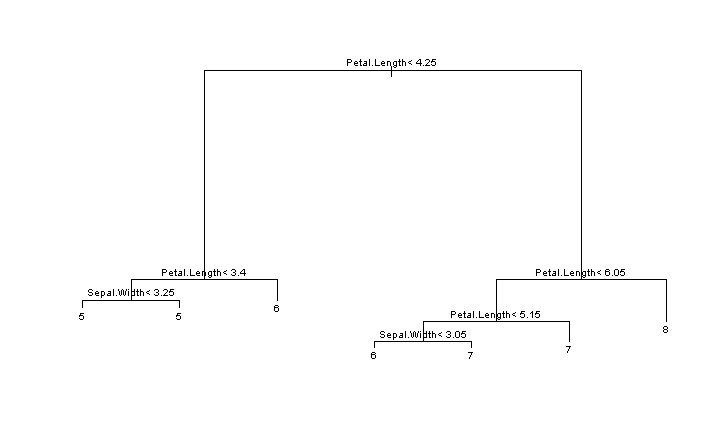If a selection of the variables in the dataset are used as predictor variables, write the function as below.

RP3 <- rpart(Species ~ Sepal.Length + Sepal.Width, iris)

You can notice the distinct difference from the above tree where other variables are used as predictors.

## n= 150
##
## node), split, n, loss, yval, (yprob)
##       * denotes terminal node
##
##  1) root 150 100 setosa (0.33333 0.33333 0.33333)
##    2) Sepal.Length< 5.45 52   7 setosa (0.86538 0.11538 0.01923)
##      4) Sepal.Width>=2.8 45   1 setosa (0.97778 0.02222 0.00000) *
##      5) Sepal.Width< 2.8 7   2 versicolor (0.14286 0.71429 0.14286) *
##    3) Sepal.Length>=5.45 98  49 virginica (0.05102 0.44898 0.50000)
##      6) Sepal.Length< 6.15 43  15 versicolor (0.11628 0.65116 0.23256)
##       12) Sepal.Width>=3.1 7   2 setosa (0.71429 0.28571 0.00000) *
##       13) Sepal.Width< 3.1 36  10 versicolor (0.00000 0.72222 0.27778) *

##      7) Sepal.Length>=6.15 55  16 virginica (0.00000 0.29091 0.70909) *

plot(RP3) text(RP3, cex = 0.8)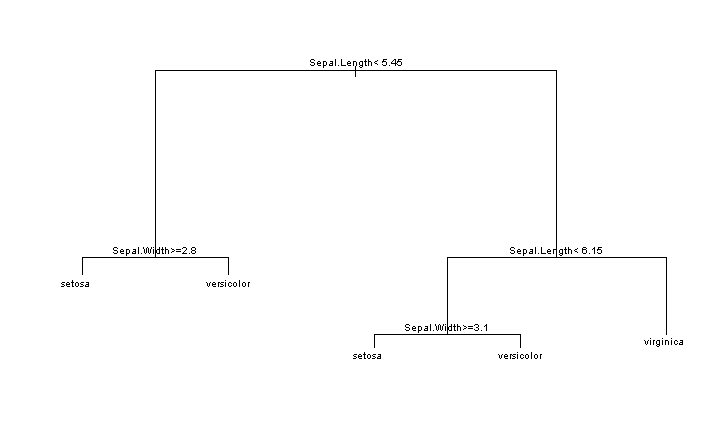### Pruning

Size of a tree often changes the accuracy of the prediction. In general, bigger tree means higher accuracy, but if the tree is too big, it overfits the data and results in decreased accuracy and robustness. By overfitting, it means the tree may be good at analysing the training data to a high degree of accuracy, but it may fail to correctly predict test data because it is grown with too much features of the training set it is not stable to any minor variations in those features. Robustness means the consistency in the performance of the tree, in this context. Hence, the objective would be to grow a tree to an optimal size that is accurate and robust enough.

Pruning is a technique used in determining the size of the tree. Often, you would grow a very large tree and starts to trim in down while measuring the change in accuracy and robustness. rpart() offers a parameter 'minsplit' which is used in this pruning process. It refers to minimum number of data points required before a split is made at a node. The below example compares the effect of changing 'minsplit' parameter.

RP4_1 <- rpart(Kyphosis ~ ., kyphosis, control = rpart.control(minsplit = 3)) RP4_2 <- rpart(Kyphosis ~ ., kyphosis, control = rpart.control(minsplit = 5)) RP4_3 <- rpart(Kyphosis ~ ., kyphosis, control = rpart.control(minsplit = 10))

par(mfcol = c(1, 3)) plot(RP4_1, main = "minsplit=3") text(RP4_1, cex = 0.7) plot(RP4_2, main = "minsplit=5") text(RP4_2, cex = 0.7) plot(RP4_3, main = "minsplit=10") text(RP4_3, cex = 0.7)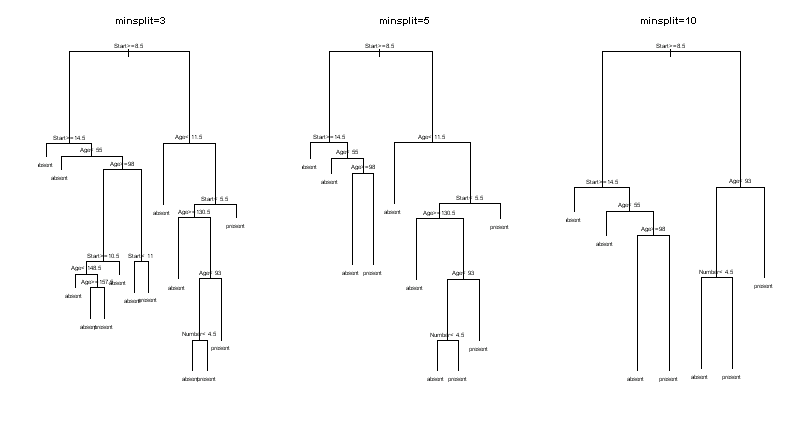Another parameter that can be used for pruning is 'complexity parameter'. This is originally created for faster growth of a tree by preventing unnecessary attempts of splits, rather than to determine the size of a tree. But, it nevertheless has impact on the size of the tree.

RP5_1 <- rpart(Kyphosis ~ ., kyphosis, control = rpart.control(cp = 0.1)) RP5_2 <- rpart(Kyphosis ~ ., kyphosis, control = rpart.control(cp = 0.001)) RP5_3 <- rpart(Kyphosis ~ ., kyphosis, control = rpart.control(cp = 1e-04))

par(mfcol = c(1, 3)) plot(RP5_1, main = "cp=0.1") text(RP5_1, cex = 0.7) plot(RP5_2, main = "cp=0.001") text(RP5_2, cex = 0.7) plot(RP5_3, main = "cp=0.0001") text(RP5_3, cex = 0.7)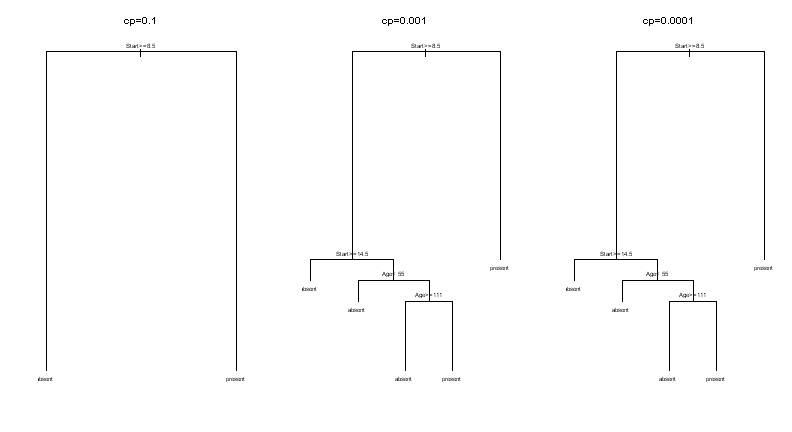### One options is to use the package 'rattle', and use the function asRules().

require(rattle)
asRules(RP5_2)
Rule number: 3 [Kyphosis=present cover=19 (23%) prob=0.58]
Start< 8.5
Rule number: 23 [Kyphosis=present cover=7 (9%) prob=0.57]
Start>=8.5
Start< 14.5
Age>=55
Age< 111
Rule number: 22 [Kyphosis=absent cover=14 (17%) prob=0.14]
Start>=8.5
Start< 14.5
Age>=55
Age>=111
Rule number: 10 [Kyphosis=absent cover=12 (15%) prob=0.00]
Start>=8.5
Start< 14.5
Age< 55
Rule number: 4 [Kyphosis=absent cover=29 (36%) prob=0.00]
Start>=8.5
Start>=14.5

Alternatively, 'rpart' package offers path.rpart() function to extract the branch of the tree to the specified node. Note that the node does not have to be final node.

For RP5_2 from the above examples that looks like the following,

RP5_2

n= 81
node), split, n, loss, yval, (yprob)
* denotes terminal node
1) root 81 17 absent (0.79012346 0.20987654)
2) Start>=8.5 62  6 absent (0.90322581 0.09677419)
4) Start>=14.5 29  0 absent (1.00000000 0.00000000) *
5) Start< 14.5 33  6 absent (0.81818182 0.18181818)
10) Age< 55 12  0 absent (1.00000000 0.00000000) *
11) Age>=55 21  6 absent (0.71428571 0.28571429)
22) Age>=111 14  2 absent (0.85714286 0.14285714) *
23) Age< 111 7  3 present (0.42857143 0.57142857) *
3) Start< 8.5 19  8 present (0.42105263 0.57894737) *

we can extract node 5 as below:
path.rpart(RP5_2,node=5)
node number: 5
root
Start>=8.5
Start< 14.5

or we can extract node 11 as below:

path.rpart(RP5_2,node=11)
node number: 11
root
Start>=8.5
Start< 14.5
Age>=55

To identify leaf nodes

FRAME<-RP5_2\$frame
FRAME[which(FRAME\$var == "<leaf>"), ]
var  n wt dev yval complexity ncompete nsurrogate    yval2.V1    yval2.V2
4  <leaf> 29 29   0    1      0.001         0               0         1.00000000   29.00000000
10 <leaf> 12 12   0    1      0.001         0              0         1.00000000   12.00000000
22 <leaf> 14 14   2    1      0.001         0              0         1.00000000   12.00000000
23 <leaf>  7  7   3    2      0.001          0               0         2.00000000    3.00000000
3  <leaf> 19 19   8    2      0.001         0               0         2.00000000    8.00000000

yval2.V3       yval2.V4      yval2.V5   yval2.nodeprob
4   0.00000000  1.00000000  0.00000000     0.35802469
10  0.00000000  1.00000000  0.00000000     0.14814815
22  2.00000000  0.85714286  0.14285714     0.17283951
23  4.00000000  0.42857143  0.57142857     0.08641975
3  11.00000000  0.42105263  0.57894737     0.23456790

To find path to the leaf node that show higher chance for the outcome 'absent' and has more than 20 cases.

sel<-FRAME[which(FRAME\$var=="<leaf>" & FRAME\$yval==1 & FRAME\$n>20),]
sel
var  n wt dev yval complexity ncompete nsurrogate   yval2.V1   yval2.V2
4  <leaf> 29 29   0    1       0.001           0             0          1.0000000   29.0000000
yval2.V3    yval2.V4    yval2.V5   yval2.nodeprob
4  0.0000000   1.0000000   0.0000000      0.3580247

path.rpart(RP5_2,rownames(sel))
node number: 4
root
Start>=8.5
Start>=14.5

### Application of a decision tree in the machine learning

The below example randomly splits the data set into training and test sets by 7:3 ratio while ensuring that both sets are mutually exclusive.

a <- sample(c(1:nrow(kyphosis)), size = nrow(kyphosis) * 0.7, replace = FALSE) Train <- kyphosis[a, ] Test <- kyphosis[-a, ]

The training set is used to build a tree. In this example, the output variable is 'Kyphosis'. As this is a categorical variable, the tree that is built here will be a classification tree.

RP <- rpart(Kyphosis ~ ., Train, control = rpart.control(minsplit = 3))

The tree may now be considered a statistical model. This model is now used to predict the output values of the test set. In this example, the output value is selected by observing the maximum probability of the output values at the node. In the real application, there will be many cross validations to determine the optimal settings and appropriate threshold point.

Pred <- predict(RP, Test[, c("Age", "Number", "Start")]) Test\$Prediction <- paste("predicted", colnames(Pred)[apply(Pred, 1, which.max)], sep = "_") table(Test\$Kyphosis, Test\$Prediction)

##           predicted_absent predicted_present
##   absent                14                 6
##   present                1                 4

The above table shows the errors of this model. In this example, the actual outputs consisted of 20 'absent' and 5 'present', and the tree predicted 15 'absent' and 10 'present'. Of the 15 predicted 'absent' 14 were correct determinations, and of the 10 predicted 'present' only 4 were correct.

If the target of this prediction is identifying 'present', then we would call the the one 'present' that was misclassified as 'absent' a False Negative, and 6 'absent' misclassified as 'present' False Positives. These error rates are used in evaluating the model performance.

#### 4 comments:

1.Hi, thanks for this blog!
What does cover=19 (23%) mean in the decision tree rules?

1.Hi Sally. Thanks for visiting.
'cover=19' means there are 19 records split into that path (branch) of the tree at that node. The example had 81 records, and when it was split at the root, 62 went to node '2' and remaining 19 went to node '3'. Hope this clarifies.

2.This comment has been removed by the author.

3.